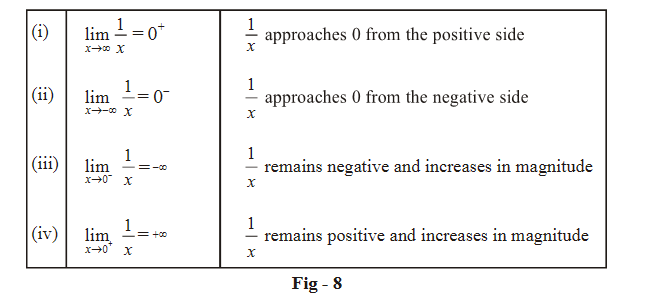# Introduction To Limits

Go back to  'LCD'

The concept of limits forms the basis of calculus and is a very powerful one. Both differential and integral calculus are based on this concept and as such, limits need to be studied in good detail.

This section contains a general, intuitive introduction to limits.

Consider a circle of radius r.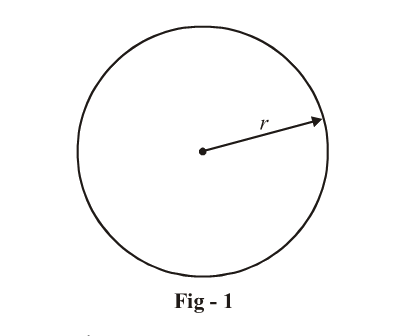We know that the area of this circle is $$\pi {r^2}$$ How?

The ancient Greeks derived this result using the concept of limits.

To see how, recall the definition of $$\pi$$ .

\begin{align}&\pi = \frac{{{\rm{length}}\,{\rm{of}}\,{\rm{circumference}}}}{{{\rm{length}}\,{\rm{of}}\,{\rm{diameter}}}}\\&\pi = \frac{c}{d} = \frac{c}{{2r}} \\&c = 2\pi r\end{align}

With this definition in hand, the Greeks divided the circle as follows (like cutting a cake or a pie):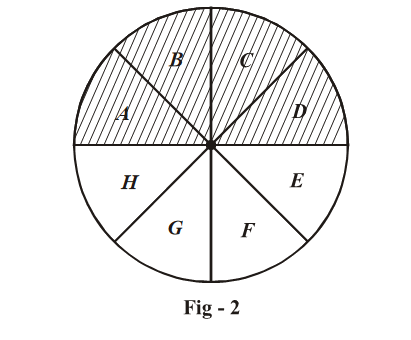Now they took the different pieces of this ‘pie’ and placed them as follows: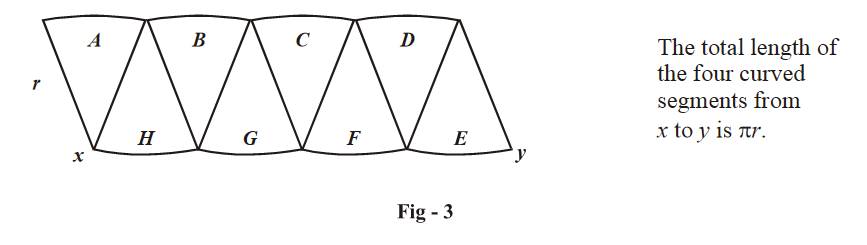See what happens if the number of cuts are increased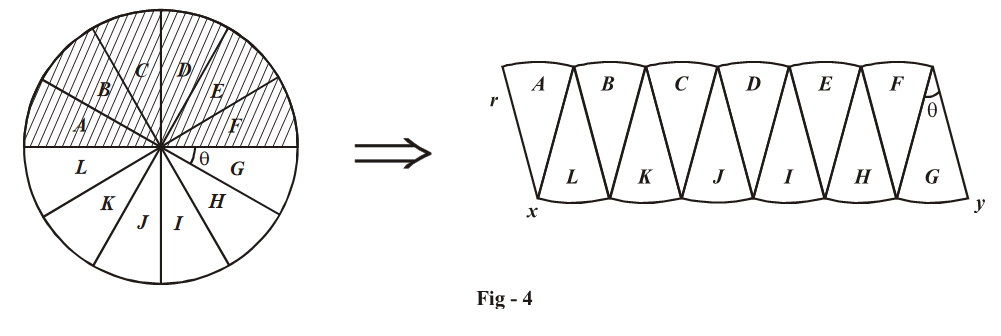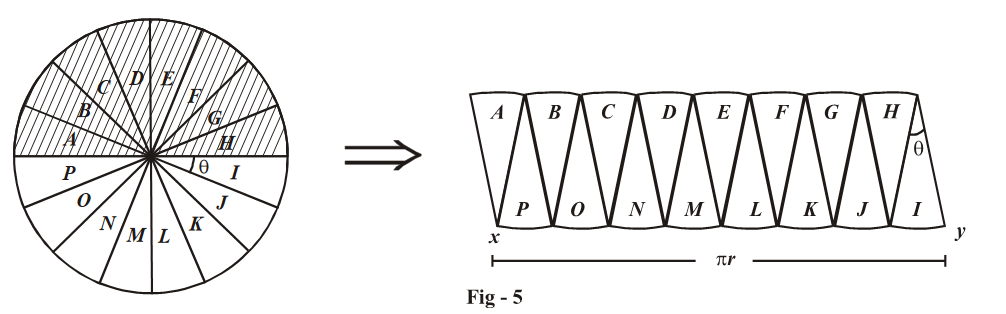The figure on the right side starts resembling a rectangle as we increase the number of cuts to the circle. The sequence of curves that joins x to y starts becoming more and more of a straight line with the same total length $$\pi r$$ .

What happens as we increase the number of cuts indefinitely, or equivalently, we decrease $$\theta$$ indefinitely? The figure ‘almost’ becomes a rectangle, though never becoming a rectangle exactly. The area ‘almost’ becomes $$\pi r \times r = \pi {r^2}.$$

In the language of limits, we say that the figure tends to a rectangle or the area A tends to $$\pi {r^2},$$ or the limiting value of area is $$\pi {r^2}.$$

In standard terminology.

$\mathop {{\rm{lim}}}\limits_{\theta \to 0} {\rm{A}} = \pi {r^2}$

Hence, we see that a limit describes the behaviour of some quantity that depends on an independent variable, as that independent variable ‘approaches’ or ‘comes close to’ a particular value.

For example, how does \begin{align}\frac{1}{x}\end{align} behave when x becomes larger and larger? \begin{align}\frac{1}{x}\end{align} becomes smaller and smaller and ‘tends’ to 0.

We write this as

$\mathop {\lim }\limits_{x \to \infty } \frac{1}{x} = 0$

How does  \begin{align}\frac{1}{x}\end{align} behave when x becomes smaller and smaller and approaches 0? \begin{align}\frac{1}{x}\end{align} obviously becomes larger and larger and ‘tends’ to infinity.

We write this as:

$\mathop {\lim }\limits_{x \to 0} \frac{1}{x} = \infty$

The picture is not yet complete. In the example above, x can ‘approach’ 0 in two ways, either from the left hand side or from the right hand side:

$$x \to {0^ - }$$ : approach is from left side of 0

$$x \to {0^ + }$$ : approach is from right side of 0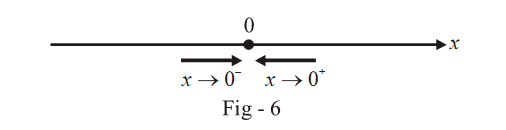How do we differentiate between the two possible approaches? Consider the graph of \begin{align}f\left( x \right) = \frac{1}{x}\end{align} carefully.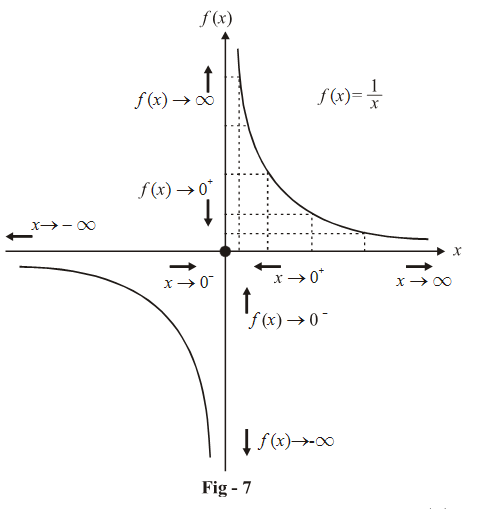As we can see in the graph above, as x increase in value or as $$x \to \infty ,\,\,f\left( x \right)$$ decreases in value and approaches 0 (but it remains positive, or in other words, it approaches 0 from the positive side)

This can be written

$\mathop {\lim }\limits_{x \to \infty } f\left( x \right) = {0^ + }$

Similarly,

$\mathop {\lim }\limits_{x \to {0^ + }} f\left( x \right) = + \,\infty$

What if x approaches 0, but from the left hand side $$\left( {x \to {0^ - }} \right)?$$ From the graph, we see that as \begin{align}x \to {0^ - },\,\,\frac{1}{x}\end{align} increases in magnitude but it also has a negative sign, that is \begin{align}\frac{1}{x} \to - \,\infty .\end{align}

What if \begin{align}x \to - \,\infty ?\,\,\,\frac{1}{x}\end{align} decreases in magnitude (approaches 0) but it still remains negative, that is, \begin{align}\frac{1}{x}\end{align} approaches 0 from the negative side or \begin{align}\frac{1}{x} \to {0^ - }\end{align}

These concepts and results are summarized below: